# Granite cube

What is the weight in kg granite cube with an edge of 0.5 m if 1dm3 of granite weight 2600 g?

Result

m =  325 kg

#### Solution:Leave us a comment of example and its solution (i.e. if it is still somewhat unclear...):

Showing 0 comments:Be the first to comment!#### To solve this example are needed these knowledge from mathematics:

Do you know the volume and unit volume, and want to convert volume units? Do you want to convert mass units?

## Next similar examples:

1. Cube cornersThe wooden cube with edge 64 cm was cut in 3 corners of cube with edge 4 cm. How many cubes of edge 4 cm can be even cut?
2. AquariumAquarium is cube with edge 45 cm. How much water can fit in there?
3. Cube basicsHow long is the edge length of a cube with volume 23 m3?
4. Volume of cubeSolve the volume of a cube with width 26cm .
5. Liter of goldWhat weight does 1 dm3 of gold have? Gold density is 19,300 kg / m3
6. AquariumAquarium is rectangular box with square base containing 76 liters of water. Length of base edge is 42 cm. To what height the water level goes?
7. Density 2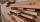What is the density of a piece of driftwood that has the mass of 25 grams and a volume is 30 cm to the 3rd power. And how do I figure it out?
8. Wood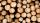Wood density is 0.6 g/cm3. How many kilograms weight 1 m3 of wood?
9. Cylindrical tank 2If a cylindrical tank with volume is used 12320cm raised to the power of 3 and base 28cm is used to store water. How many liters of water can it hold?
10. Solid in waterThe solid weighs in air 11.8 g and in water 10 g. Calculate the density of the solid.
11. SnowSnow fell overnight layer of thickness 19 cm. In the morning I had to clear a path 69 m long and one meter wide. How many cubic meters of snow I clear? How many kilos was it? (1 m3 fresh snow weighs 350 kg)
12. Tons of coal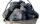Coal hopper has a capacity of 285 liters. How many tons is it? The bulk density of coal is 916 kg/m3.
13. Excavator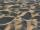The excavator bucket capacity is 0.5 m3. Determine the mass of sand that the excavator picks up. The sand density is 1650 kg/m3.
14. 3 children3 children had to divide 4 pounds is candy. How much candy did each child get?
15. The shopThe shop has 3 hectoliters of water. How many liter bottles is it?
16. Infants water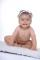From the tank, which has 10 hectoliters of water for infants, went 580 liters at mornening and 340 liters in the afternoon. How many liters left in the tank?
17. Addition of Roman numbersAdded together and write as decimal number: LXVII + MLXIV